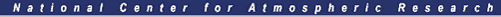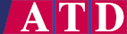#SHEBA ISFF Flux-PAM Project Report

### 10.0 Data Processing and Data Archives

#### 10.1 Five-Minute Data

The SHEBA Flux-PAM data are available at two time resolutions, five-minute averages and one-hour averages.T. Uttal
The basic data archived during field operations are five-minute averages calculated at each Flux-PAM site by the EVE data systems. The five-minute data were stored on removable media on the local station data systems and also transmitted by RF modem to the base computer on the Des Grosseiliers. These two overlapping data sets have been merged during post-project processing to fill in non-coincident data gaps present in both sets. Table 10.1 lists the five-minute data variables measured directly by the Flux-PAM stations, as well as the one-hour-averaged variables.

Various corrections have been applied to the five-minute data during post-project processing. Most of these have been discussed in the sections of the report that correspond to individual sensors. They include

• interpolating between pre- and post-project radiometer calibrations,
• correction to the compass reading for offsets generated when the beacon was on,
• use of a 1-hour median filter to remove known spikes in the compass and hygrothermometer data in October 1997,
• subtracting offsets from the radiometer level data,
• calibration correction to the Tsoil/ice sensor at Station 2 after April 11,
• correction to Solent virtual temperature data prior to June 21,
• where possible, the wind measurements from the sonic anemometer have been rotated into a coordinate system with its vertical axis normal to a plane defined by the local mean wind field.

Missing or obviously erroneous compass data have not been replaced in the 5-minute data record (with the exception noted above), but obviously erroneous data have been flagged as missing, including compass data collected during operation of the sonic heaters. For most of the project, the orientation of the stations, and thus the compass data, varied rather slowly. Thus it was thought to be generally acceptable to fill in the missing data by interpolation (and extraplotion) during the calculation of derived variables such as Vazimuth, dir, or boom.

Table 10.1 Flux-PAM Data Variables
Name Variable, units Limits 5-min 1-hour
P pressure, mb 970, 1050 x x
T.plat PRT temperature, °C   x
T.therm thermistor temperature, °C   x
T air temperature, °C -45, 5   x
RH relative humidity wrt water, %RH   x
RHice relative humidity wrt ice, %RH 60, 115   x
Rsw.in incoming shortwave radiation, W/m⊃2 -15, 900 x x
Rsw.out outgoing shortwave radiation, W/m⊃2 -15, 900 x x
Rpile.in,out pyrgeometer thermopile, W/m⊃2   x
Tcase.in,out pyrgeometer case temperature, °C   x
Tdome.in,out pyrgeometer dome temperature, °C   x
Rlw.in incoming longwave radiation, W/m⊃2 100, 350   x
Rlw.out outgoing longwave radiation, W/m⊃2 150, 350   x
Rlwdiff.in.ARM,
ETL
NCAR - ARM(ETL), W/m⊃2
x
Tsoil, Tice** ice temperature, °C -25, 5 x x
Gsoil, Gice** ice heat flux, W/m⊃2 -125, 100 x x
u,v horizontal velocities, m/s 0, 17 x
spd wind speed, m/s 0, 17   x
dir wind direction wrt true N, m/s 0, 360   x
w vertical velocity, m/s 0, 0.5 x
tc sonic virtual temperature, °C   x
u'u' turbulent variance, m²/s² 0, 4* x x*
u'v' turbulent covariance, m²/s² ± 0.5* x x*
u'w' turbulent covariance, m²/s² ± 0.6* x x*
u'tc' turbulent covariance, °C-m/s ± 0.3* x x*
v'v' turbulent variance, m²/s² 0, 2.5* x x*
v'w' turbulent covariance, m²/s² ± 0.3* x x*
v'tc' turbulent covariance, °C-m/s ± 0.1* x x*
w'w' turbulent variance, m²/s² 0, 0.8 x x
w'tc' turbulent covariance, °C-m/s ± 0.08 x x
tc'tc' turbulent variance, °C² 0, 0.1 x x
uflux momentum flux, Nt/m²     x
H heat flux, W/m²     x
freq.sonic sonic pulses/second per path   x
u,v,wsamples sonic pulses per sample, n   x
samples.sonic percentage of good data, % 0, 100   x
u,v,w,tcflag software-detected data outliers, n   x
Vazimuth azimuth of sonic v axis wrt N, ° 0, 360 x
boom azimuth of sonic boom wrt N, ° 0, 360 x x
heater fraction of time heater is turned on   x
latitude latitude, decimal degrees 74, 81   x
longitude longitude, decimal degrees -169, -142   x
lat,long.deg latitude, longitude degrees   x
lat,long.min latitude, longitude minutes   x
lat,long.sec latitude, longitude seconds   x
compass compass reading, decimal degrees   x
beacon fraction of time beacon is turned on   x
Tbox.eve electronics internal temperature, °C   x
vdc.batt battery voltage, V   x
vdcmax,min.batt max, minimum battery voltage, V   x
vdc.teg system voltage, V   x
vdcmax,min.teg max, minimum system voltage, V   x
i.charge battery charging current, amps   x
imax.charge max charging current, amps   x
Z.TRH.meas TRH sensor height above snow, interpolated from direct measurements, meters   x***
Z.TRH.snowline TRH sensor height above snow, interpolated using snow-line data, meters    x***
Z.sonic.meas sonic anemometer height above snow, interpolated from direct measurements, meters    x***
Z.sonic.snowline sonic anemometer height above snow, interpolated using snow-line data, meters    x***
* streamwise coordinates
** hour averages
*** provided by Ed Andreas, Keran Claffey (see appendix)

#### 10.2 One-Hour Data

Hourly averages of most data parameters, centered on the half-hour, have been calculated during post-project processing. The hour-average parameters generally, but not always, correspond to the five-minute parameters. For example, the pyrgeometer thermopile and temperature measurements have been combined to calculate the long-wave radiation flux, the PRT and thermistor data have been combined into a single value for the air temperature, and the PRT and RH (with respect to water) data have been combined to calculate the relative humidity with respect to ice.

Prior to calculating hour averages, two editing steps were applied to the data to minimize the contribution of occasional erroneous spikes. First absolute range limits, listed in Table 10.1, were imposed on the data. Observations exceeding these limits were flagged as missing. Second, a running 3-point (15 minute) median filter was applied to the five-minute time series, which replaced the central data point with the median of the data within the 15-minute window. If the data within the 15-minute window are monotonically increasing or decreasing (or constant), they are unaffected by the median filter. However, valid maxima or minima within the window are suppressed in the same manner as erroneous spikes, reducing the variance of the five-minute time series. Reduction of the five-minute variance is not expected to have a significant effect on the hour average.

#### 10.3 Processing of Sonic Anemometer Data

Most parameters have been sampled by the EVE data system at a rate of 1 Hz, while the sonic anemometer data have been ingested at a rate of 20.83 Hz for the R2 Solents and 10 Hz for the ATIs. In order to determine turbulent variances and fluxes from the high-rate sonic data, EVE calculated and archived a complete set of centered 5-minute covariances:



During data post-processing, these covariances were rotated into a coordinate system with its vertical (w) axis normal to the plane of the mean flow. However the (five-minute) data in the horizontal plane determined by that rotation remain in a coordinate system nominally aligned with the sonic measurement array. The data may be rotated about the vertical axis into a geographic coordinate system aligned with true North using the variable Vazimuth.

The hour-averaged sonic data were calculated with the following steps:

1. Five-minute mean and covariance data are flagged as missing if samples.sonic is less than 99.5% or heater is non-zero (sonic heaters on during the period)
2. Compute one-hour vector-mean wind speed and direction
3. Rotate five-minute means and covariances to one-hour streamwise coordinates
4. Apply range limits and median filter to five-minute covariances
5. Compute 15-minute covariances, including covariance of 5-minute means (see below)
6. Average four 15-minute covariances to obtain hour averages
Thus the hour-average covariances are high-pass filtered at an effective period of 15 minutes. The 15-minute covariances account for all fluctuations at shorter periods by including the covariance of the 5-minute means in Step 5 as, e.g.

<u'w'>15 = M15(<u'w'>) + M15(<u><w>) - M15(<u>)M15(<w>)

where <u'w'>15 is the 15-minute covariance, <u> and <w> are 5-minute means, <u'w'> is a 5-minute covariance, and M15(x) is the average of three 5-minute values of x.

The hour-averaged data also include the calculation of momentum and heat fluxes,

uflux = rho M60(<u'w'>15)

H = rho CP M60(<w'tc'>15)

where M60(x15) is the average of four 15-minute values of x. Daily average values of air density rho and the specific heat of air CP were used in these calculations, in order to assure that they were immune to the possiblity of errors in the individual 5-minute values of temperature, pressure and humidity. Note also that the heat flux is calculated using the sonic virtual temperature rather than true air temperature.

Note that the sonic data have not been edited for possible flow distortion when the mast is upwind of the sonic. The mast is upwind of the sonic when dir = boom ± 180°. This is particularly an issue for the ATI sonics, which were mounted at the same height as the sonic boom and thus below the top of the vertical mast. However it also may be an issue for the asymmetric R2A Solents. Although the Solents where mounted above the boom and above the top of the vertical mast, the three vertical struts supporting the R2A sonic array are clustered within a 120° sector which was usually oriented toward the mast.